# XPath Axes: Ancestor, Following Sibling, Preceding

XPath axes in Selenium are methods to identify those dynamic elements which are not possible to find by normal XPath method such as ID, Classname, Name, etc.

Axes are so named because they tell about the axis on which elements are lying relative to an element.

Dynamic web elements are those elements on the webpage whose attributes dynamically change on refresh or any other operations.

The commonly useful XPath axes methods used in Selenium WebDriver are child, parent, ancestor, sibling, preceding, self, namespace, attribute, etc.

XPath axes help to find elements based on the element’s relationship with another element in an XML document.

XML documents contain one or more element nodes. They are considered as trees of nodes.

If an element contains content, whether it is other elements or text, then it must be declared a start tag and an end tag.

The text defined between the start tag and end tag is the element content.

The topmost element of the tree is called root element.

An example of a basic HTML page is shown below screenshot.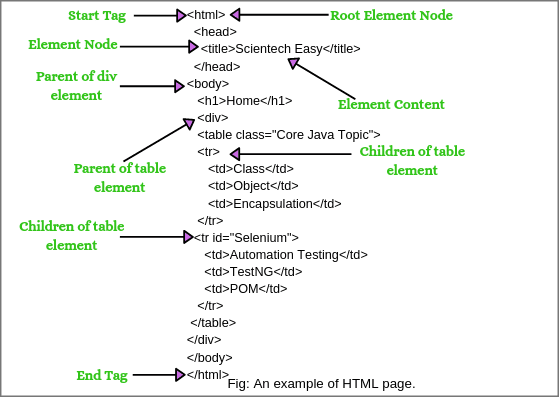Let’s understand some basic XPath terminology before moving XPath axes.

## XPath Terminology: Nodes, Atomic values, Parents, Children, Siblings

1. Nodes:

DOM represents trees of nodes. The topmost element of the tree is called root node. The example of nodes in the XML document above:

“html” is the root of element node, “title” is an element node. XPath contains seven kinds of nodes: element, attribute, text, namespace, processing-instruction, comment, and document nodes.

2. Atomic values:

The node which has no parents or children is called atomic values. For example, Automation Testing, TestNG, POM.

3. Parents:

Each element and attribute has one parent like father or mother. For example, “body” is the parent of div element. “div” is the parent of the table element.

4. Children:

Element nodes that may contain zero, one, or more children. For example, tr is children of table element, div is the children of body element, table is children of div element.

5. Siblings:

The node that has the same parent is called siblings. In the above XML document, title and body elements both are siblings.

## XPath Axes Methods in Selenium

To navigate the hierarchical tree of nodes in an XML document, XPath uses the concept of axes. The XPath specification defines a total of 13 different axes that we will learn in this section.

A list of 13 XPath axes methods in Selenium WebDriver is as follows:

1. Child Axis
2. Parent Axis
3. Self Axis
4. Ancestor Axis
5. Ancestor-or-self Axis
6. Descendant Axis
7. Descendant-or-self Axis
8. Following Axis
9. Following-sibling Axis
10. Preceding Axis
11. Preceding-sibling Axis
12. Attribute Axis
13. Namespace Axis

Each axis contains various nodes that depend on the current node. An XPath axis is a collection of nodes that satisfy the current navigation criteria.

## Child Axis:

The child axis is one of the 13 XPath axes that contains all the child nodes of the current context. It selects all children elements of the current node.

The syntax of child axis is given below:

```Syntax:
//child::tagName```

For example, open webpage https://www.yandex.com, right-click on Yandex.in and go to inspect option as shown below screenshot.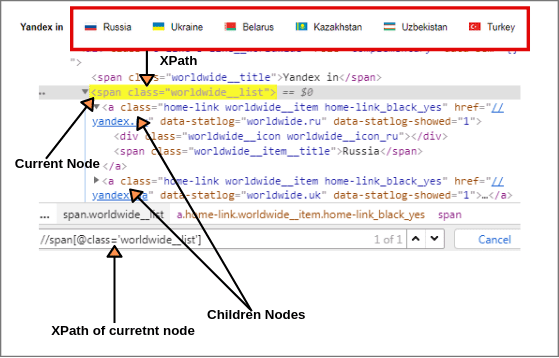Select all children elements of the current node as shown in the above screen. First, we will find XPath of the current node.

`XPath of current node: //span[@class = 'worldwide__list']`

Now we will find out XPath of children elements of current node using child axis as shown in above figure.

`XPath of all children elements: //span[@class = 'worldwide__list']//child::a (1 of 6 matched)`

This expression identified six children nodes using the child axis. We can get the XPath of different children elements according to the requirement by putting ,…………and so on.

```XPath(Russia): //span[@class = 'worldwide__list']//child::a  (1 of 1 matched)

XPath(Ukraine): //span[@class = 'worldwide__list']//child::a (1 of 1 matched)

XPath(Belarus): //span[@class = 'worldwide__list']//child::a and so on.```

## Parent Axis:

The parent axis selects the parent of the current node. The parent node may be either root node or element node. The root node has no parent.

Therefore, when the current node is root node, the parent axis is empty. For all other element nodes, the parent axis contains a maximum of one node.

The syntax of parent axis is given below:

```Syntax:
//parent::tagName```

Let’s take a scenario for example.

Open the website yandex.com and right-click on the search box. We will find the parent of the current node. Choose a search input box as a current node and find XPath of the current node (Search box).

`XPath(Current node): //input[@id = 'text'] (1 of 1 matched).`

Now we will find the XPath of the parent element node of current node using parent syntax as shown below screenshot: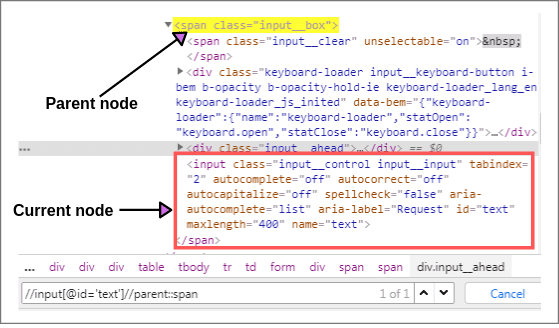`XPath(Parent node): //input[@id = 'text']//parent::span (1 of 1 matched)`

It will select the parent node of the input tag of Id = ‘text’.

`XPath(Parent node): //input[@id = 'text']//parent::* (1 of 1 matched)`

## Self Axis:

Self axis selects the element of the current node. It always finds only one node that represents self-element.

```Syntax:
//self::tagName```

For example, we can also find XPath of the current element node using self axis as shown in the above screenshot.

```XPath(Current node): //input[@id = 'text']//self::input (1 of 1 matched)

or,

XPath(Current node): //input[@id = 'text']//self::*```

## Ancestor Axis:

The ancestor axis selects all ancestor elements (parent, grandparent, great-grandparents, etc.) of the current node. This axis always contains the root node (unless the current node is the root node).

```Syntax:
//ancestor::tagName```

Let’s take an example to understand the concepts of ancestor axis.

Open www.facebook.com, right-click on the login button, and go to inspect option. You will see HTML code of login button as shown below screenshot: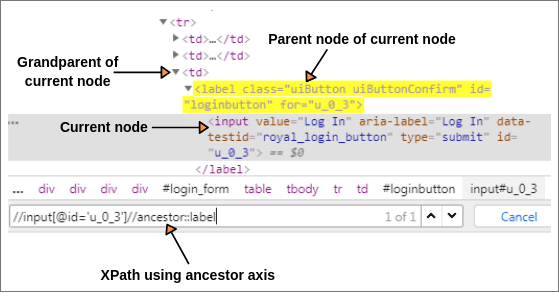Let us consider the login button as current node. First, we will find the XPath of current node.

`XPath(Current node): //input[@id = 'u_0_a']`

Now, we will find XPath of parent and grandparent of current node.

```XPath(Parent node): //input[@id = 'u_0_a']//ancestor::label

XPath(Grandparent node): //input[@id = 'u_0_a']//ancestor::td```

In both cases, 1 of 1 node is matched by using “ancestor” axis.

## Ancestor-or-self Axis:

This axis selects all ancestor elements (parent, grandparent, great-grandparents, etc.) of the current node and the current node itself.

Let us find XPath of current node (login button) by using the ancestor-or-self axis.

`XPath(login button): //input[@id = 'u_0_3']//ancestor-or-self::input`

The above expression identified the current element node.

## Descendant Axis:

The descendant axis selects all descendant elements (children, grandchildren, etc) of the current node. Let’s take an example to understand the concepts of the descendant axis.

Open webpage https://pixabay.com/accounts/register/?source=signup_button_header, right-click on Username element, and go to inspect option.

As shown in the below screenshot, let’s suppose “signup_form new” as a current node. You can bring the cursor to this node to see current node.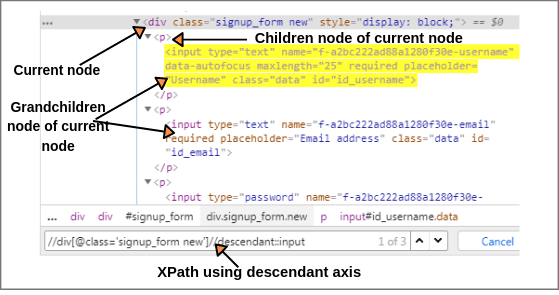The XPath of current node will be as follow:

`XPath(Current node): //div[@class = 'signup_form new']`

Now using the descendant axis with above XPath, we can find easily all children, grandchildren elements, etc of current node.

`XPath: //div[@class = 'signup_form new']//descendant::input (1 of 3)`

The above XPath expression identified three elements like username, password, and email address. So, we can write XPath by putting 1, 2, and 3 in the above expression.

```XPath(Username): //div[@class='signup_form new']//descendant::input (1 of 1 matched)

XPath(Email address): //div[@class = 'signup_form new']//descendant::input

```

## Descendant-or-self Axis:

The descendant-or-self axis selects all descendants (children, grandchildren, etc) of the current node and current node itself. In the above screenshot, div is the current node. We can select this current node using the descendant-or-self axis.

The XPath of current node is as follows:

`XPath(Current node): //div[@class = 'signup_form new']//descendant-or-self::div`

The above expression identified 1 node. If we change tagname in the above XPath expression from div to input then we can get node of username, email address, and password.

Let’s find the XPath of these nodes.

```XPath(Username): //div[@class = 'signup_form new']//descendant-or-self::input

XPath(Email address): //div[@class = 'signup_form new']//descendant-or-self::input```

## Following Axis:

The following axis selects all elements (nodes) in the document after closing tag of the current node. Let’s consider “First name” input box as current node in the Facebook webpage.

The XPath of the current node is as follows:

`XPath(Current node): //input[@id = 'u_0_r']`

Now we will find all elements like Surname, Mobile number, etc by using the following axis of the current node. The below syntax will select the immediate node following the current node.

`XPath: //input[@id = 'u_0_r']//following::input (1 of 23)`

The above expression has identified 23 nodes matching by using “following” axis-surname, mobile number, new password, etc.

If you want to focus on any particular element then you can change the XPath according to the requirement by putting ,…………and so on like this.

`XPath(Surname): //input[@id = 'u_0_r']//following::input (1 of 1 matched)`

By putting “1” as input, the above expression finds the particular node that is ‘Surname’ input box element.

Similarly, on putting “2” as input,

`XPath(Mobile number): //input[@id = 'u_0_r']//following::input (1 of 1 matched).`

## Following-sibling Axis:

The following-sibling selects all sibling nodes after the current node at the same level. i.e. It will find the element after the current node.

For example, the radio button of female and female text both are siblings on the Facebook home page as shown in the below screenshot.So, we will find XPath of current node i.e. XPath of the female radio button.

`XPath(Radio button): //input[@id = 'u_0_5']`

Using the following-sibling axis, we can easily find XPath of text “Female” at the same level.

`XPath(Female): //input[@id = 'u_0_5']//following-sibling::label (1 of 1 matched).`

The above expression identified one input nodes by using “following-sibling” axis.

## Preceding Axis:

The preceding axis selects all nodes that come before the current node in the document, except ancestor, attribute nodes, and namespace nodes.

Let us consider the login button as current node on the Facebook web page as shown below screenshot.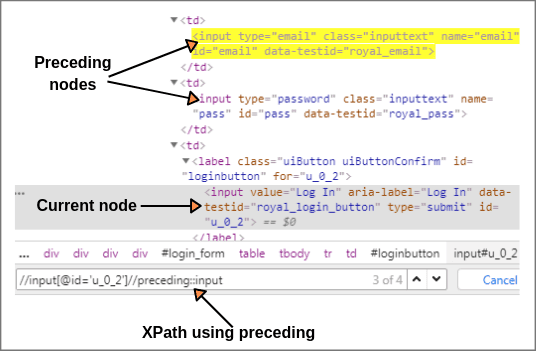Let’s first, find the XPath of current node i.e XPath of login button.

`XPath(Current node): //input[@id = 'u_0_2']`

Now we will select all nodes by using the preceding axis in the document that comes before the current node.

`XPath: //input[@id = 'u_0_2']//preceding::input (1 of 4 matched).`

The above expression identified all the input elements before “login” button using the preceding axis. 2 of 4 matches nodes are matched with Email and Password input elements.

If you want to focus on any particular element like “Email” or “Password” then you can change the XPath according to the requirement by putting ,…………and so on. For example:

```XPath(Email): //input[@id = 'u_0_2']//preceding::input (1 of 1)

## Preceding-sibling Axis:

The preceding-sibling axis selects all siblings before the current node. Let’s take an example to understand the concept of the preceding-sibling axis.

Open web page www.pixabay.com, right-click on videos link and go to inspect option.

Let’s consider videos link as current node as shown in below screenshot and find the XPath of current node by using text() method.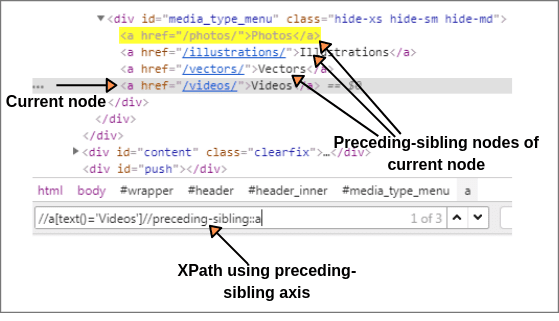`XPath(Current node): //a[text() = 'Videos']`

Now we will find all nodes using preceding-sibling axis of the current node in the document.

`XPath: //a[text() = 'Videos']//preceding-sibling::a (1 of 3)`

The above expression identified three nodes before the current node (videos link) as shown in above screenshot.

Using this expression, we can easily find XPath of preceding-sibling elements like Photos, Illustrations, and Vectors like this:

```XPath(Photos): //a[text() = 'Videos']//preceding-sibling::a

XPath(Illustrations): //a[text() = 'Videos']//preceding-sibling::a

XPath(Vectors): //a[text() = 'Videos']//preceding-sibling::a```

## Attribute Axis:

This axis selects the element node on the basis of the attribute identifier (@) of the current node. If the current node is not an element node, this axis is empty. The expressions attribute::type and @type both are equivalent.

For example:

Open the webpage www.pixabay.com, right-click on the search input box, and go to inspect. We can write the XPath of search input box (current node) using the attribute axis.

`XPath(Search box): //input[attribute::name = 'q']`

## Namespace Axis:

The namespace axis is one of 13 XPath axes that selects all namespace nodes associated with current node. If the current node is not an element node then this axis will be empty.

Hope that this tutorial has covered almost all important points related to 13 XPath axes methods with examples. I hope that you will have understood and enjoyed this topic and performed it in the chrome browser.

The XPath axes like child, parent, ancestor, following, and preceding are vary important for the Selenium technical test in any company.